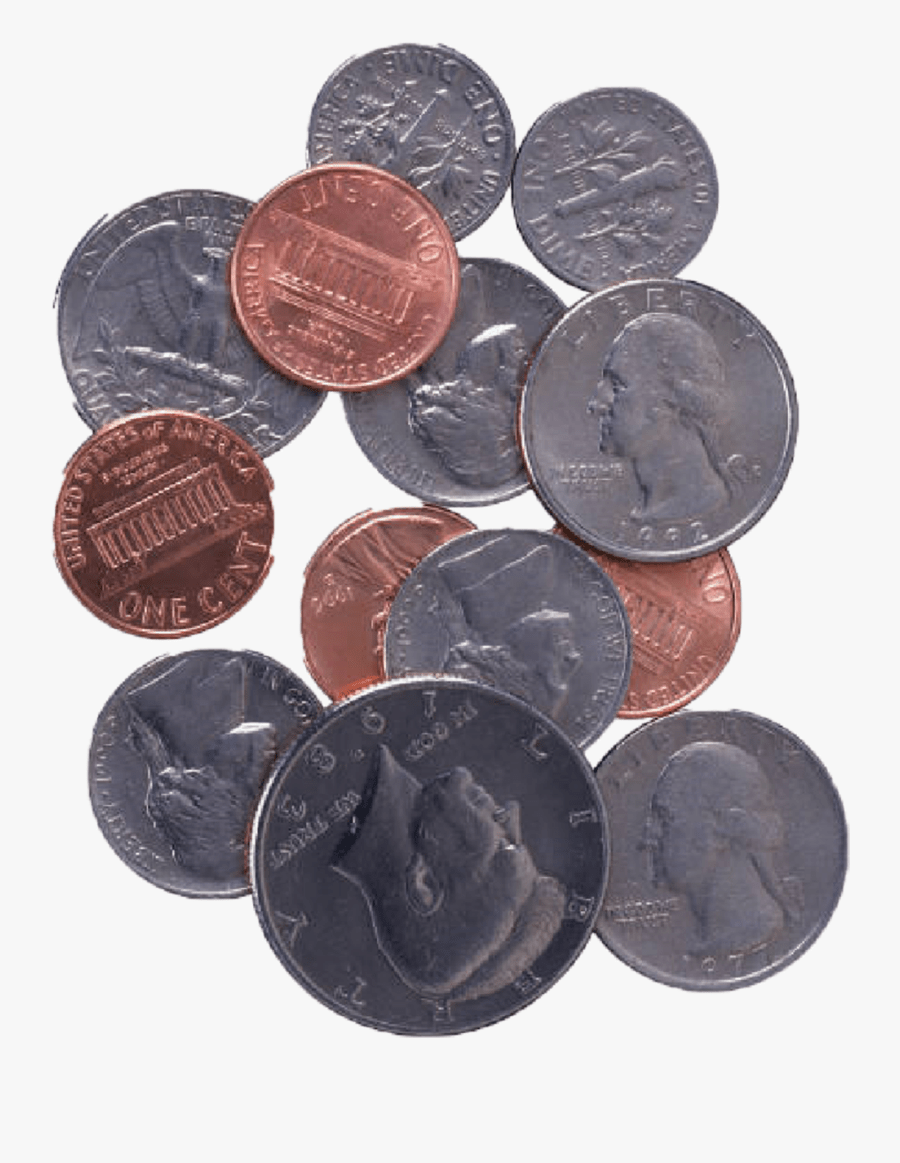Subtraction
Geometry
Fractions
Money Math
100

4+8=

12

100

4-0=

4

100

What is a shape with 4 equal sides and corners?

A square

100

How many quarters makes one?

4

100

How many dimes makes 10 cents?

1

200

29+6=

35

200

13-8=

5

200

Name a shape with no corners

A circle

200

How many quarters make one HALF?

2

200

How many quarters make a half dollar?

2

300

42+63=

105

300

39-16=

23

300What is this shape called?

A pyramid

300

What is 2/3 + 2/3?

4/3 or 1 1/3

300

How much does 12 quarters, 3 dimes, and 4 nickels make?

\$3.50

400

170+35=

205

400

540-25=

515

400

What shape has 7 sides?

A heptagon

400

Simplify 5/4

1 1/4

400

How many pennies make \$2.05?

205

500

528+472=

1,000

500

9,526-426=

9,100

500What is the name of this shape?

A cylinder

500

Add 5/4 + 4/5

41/20 or 2 1/20

500What is the sum of all the change?

\$1.64

Click to zoom